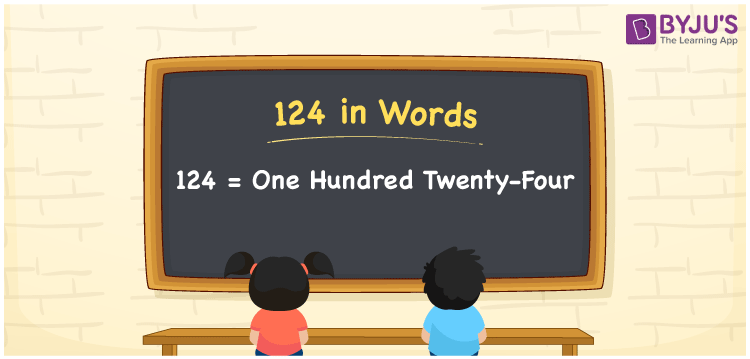# 124 in Words

124 in words is written as One Hundred Twenty-four. The name of number 124 in English is “One Hundred Twenty-four”. The word One Hundred Twenty-four is used in many real-life situations. For example, there are One Hundred Twenty-four students in a classroom. Thus, it is a counting number. Also, to represent the currency equal to 124, we can write it in words as Rupees One Hundred Twenty-four or One Hundred Twenty-four rupees. Therefore, it is necessary to learn the numbers in words, for the ease of understanding and expressing them.

 124 in words One Hundred Twenty-four One Hundred Twenty-four in Numbers 124

## 124 in English Words## How to Write 124 in Words?

If we know the place value of digits of 124, then we can easily express it in words. The place value is basically the position of a digit in a number. 124 is a three-digit number, therefore, we can specify the position of each digit of 124 in a place value chart. In the Indian numbering system, the order of place value of digits from right to left is given by:

 Hundreds Tens Ones 1 2 4

We can write it in expanded form as:

1 × Hundred + 2 × Ten + 4 × One

= 1 × 100 + 2 × 10 + 4 × 1

= 100 + 20 + 4

= 124

= One Hundred Twenty-four

Therefore, 124 in words is written as eight hundred thirties.

124 is a natural number that precedes 125 and succeeds 123.

124 in words – One Hundred Twenty-four

Is 124 an odd number? – No

Is 124 an even number? – Yes

Is 124 a perfect square number? – No

Is 124 a perfect cube number? – No

Is 124 a prime number? – No

Is 124 a composite number? – Yes

## Frequently Asked Questions on 124 in Words

Q1

### How to write 124 in words?

124 in English is written as “One Hundred Twenty-four”.
Q2

### Is 124 an odd number or even number?

124 is an even number because it is wholly divisible by 2.
Q3

### Write One Hundred Twenty-four in numbers.

One Hundred Twenty-four in numbers is 124.